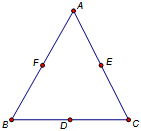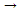﻿ Objects > Iterations and Iterated Images > How to Construct a Sierpiński Gasket

# How to Construct a Sierpiński Gasket

Navigation:  Objects > Iterations and Iterated Images >

# How to Construct a Sierpiński GasketBecause iteration can be applied to any type of Sketchpad construction, the options that support it may at first seem complex. The best way to develop an understanding of iteration is to work through examples. In this example, you’ll use iteration to define a fractal known as the Sierpiński gasket. This fractal is the limit of the process of replacing a triangle with three smaller interior triangles, one in each corner of the original triangle. Each of these three smaller triangles is replaced with three even smaller triangles; and so on. Since at each stage you are replacing a pre-image triangle with three different image triangles, you’ll need three mappings to define the fractal.1.In a new sketch, use the Segment tool to constructABC.

2.Construct the midpoints of your triangle’s edges. Use the Text tool to label the vertices A, B, and C, and the midpoints D, E, and F, as in the illustration.

This is your pre-image triangle. Note that if you constructed the three corner triangles,AFE,FBD, andEDC, each of them would be similar to the pre-image and half its size.

3.A, B, and C, and choose

4.In the Iterate dialog box, map AF, BB, and CD.

This maps the original triangle to the lower-left cornerFBD. You should see a series of triangles iterating into the lower-left corner of your original triangle.

Note that in this step you map B to itself, as this vertex is the same in both the original triangle and in the lower-left corner triangle.

5.Use the Structure pop-up menu to add a new mapping to your iteration rule. In the new mapping, map AE, BD, and CC.

This iterates your pre-image triangle to the lower-right corner, while simultaneously — by the previous map — iterating each image to the lower-left corner.

6.Use the Structure pop-up menu again to add a third and final mapping to your iteration rule. In this third mapping, map AA, BF, and CE.

This iterates your previous mappings toward the upper corner of the triangle.

7.Click Iterate to dismiss the dialog box.Be careful not to increase the number of iterations too quickly. Because each iteration adds three times as many new triangles as the previous iteration, the construction quickly becomes very complex! Sketchpad will start to slow down if your sketch contains iterations more complex than your computer can handle gracefully.

You can increase or decrease the number of displayed iterations by selecting any of the iterated images and pressing the + or key on the keyboard.

If you could iterate an infinite number of times, this process would result in a Sierpiński gasket. If you imagine the area of your initial triangle as having been replaced by the area of three smaller triangles at each step, think for a moment about what happens to the total area of all of the smaller triangles as you increase the number of iterations. Because the three smaller triangles didn’t cover the initial triangle, the area must be getting smaller. So with each iteration the area of the new triangles becomes smaller. What’s the limit of the area? How do you know? What happens to the perimeter? Fractals frequently give rise to surprising properties.

You can visualize the areas by repeating the above steps in a new sketch, using aABC in which you have used Construct | Triangle Interior to construct the triangle interior. When you’re done specifying your three mappings, choose Final iteration only from the Display pop-up menu of the Iterate dialog box. After you close the dialog box, select and hide the original interior ofABC. Also hide the iterated images of the three sides of the triangle. (The iterated images of the three vertices are normally already hidden.)

You’re left with only the iterated images of the triangle interior, so you can see how changing the depth of iteration affects the total area.